Aa Bb Cc Dd Ee Ff Gg Hh Ii Jj Kk Ll Mm Nn Oo Pp Qq Rr Ss Tt Uu Vv Ww Xx Yy Zz

Ff

Fibonacci numbers

• the Fibonacci numbers are a sequence of numbers
named after Leonardo Fibonacci,
an Italian mathematician,
who lived 1175 to 1250.
• in a Fibonacci sequence each number
is the sum of the two numbers before it
n1 + n2 = n3.

0, 1, 1, 2, 3, 5, 8, 13, 21, 34, 55, 89, 144, ...

EXAMPLES: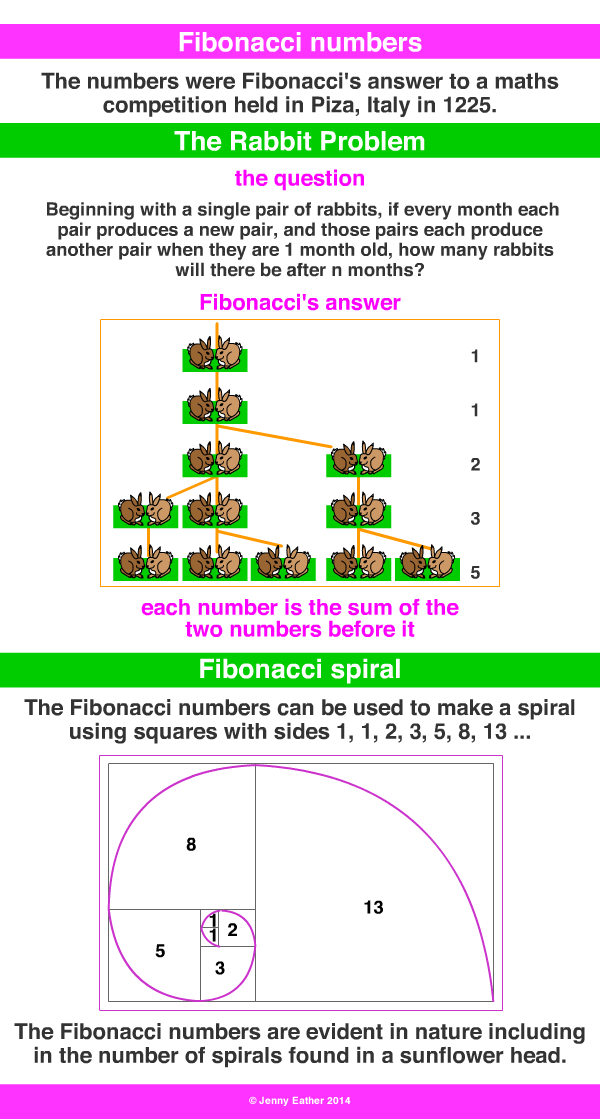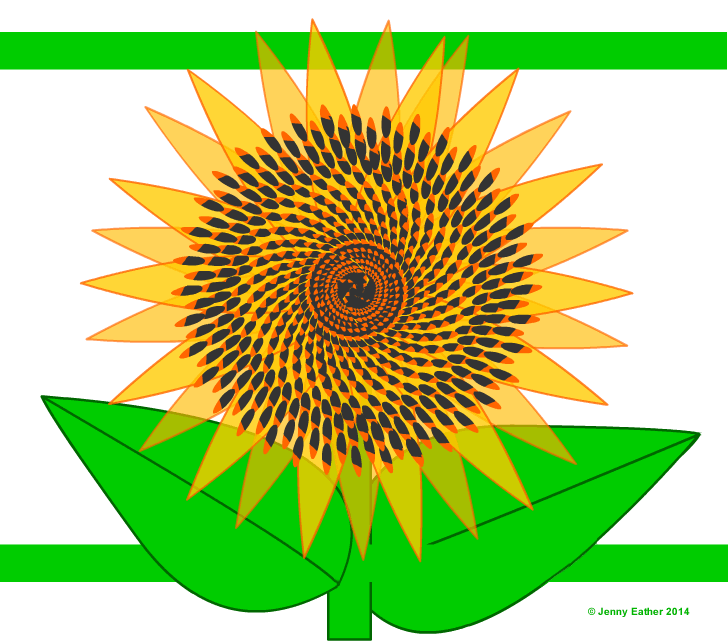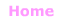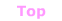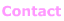© Jenny Eather 2014. All rights reserved.